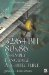Vector Addition and Subtraction (Fixed Point)For most of the number crunching in your games or tools you will most likely use single-precision floating-point. For artificial intelligence (AI) and other high-precision calculations, you may wish to use the higher precision double-precision, but it only exists in scalar form on the FPU, except for the case of the SSE2 or above, so functionality must be emulated in a sequential fashion whenever possible. But even with the higher precision, there is still a bit of an accuracy problem.

An alternative would be to use integer calculations in a fixed-point format of zero or more places. If the data size is large enough to contain the number, then there is no precision loss!

Pseudo Vec

These can get pretty verbose, as for fixed-point (integer) addition there would be support for 8-, 16-, and 32-bit data elements within a 128-bit vector and these would be signed and unsigned, with and without saturation. The interesting thing about adding signed and unsigned numbers , other than the carry or borrow , is that the resulting value will be exactly the same and thus the same equation can be used. This can be viewed in the following 8-bit example:

Unsigned

Hex

Signed

95
+ 240
335
C=1 79

05Fh
+ 0F0h
C=1 04Fh
C=1 (79)

95
+ 16
C=0 79
C=0 79

Notice that the resulting bits from the 8-bit calculation are all the same. Only the carry is different and the resulting bits are only interpreted as being signed or unsigned.

Pseudo Vec (x86)

Now let's examine these functions closer. MMX and SSE2 have the biggest payoff, as 3DNow! and SSE are primarily for floating-point support.

mov    ebx,pbB    ; Vector B mov    eax,pbA    ; Vector A mov    edx,pbD    ; Vector Destination

The following is a 16—8-bit addition but substituting a PSUBB for the PADDB will transform it into a subtraction.movq    mm0,[ebx+0]    ; Read B Data {B  7  ...B   } movq    mm1,[ebx+8]    ;             {B  F  ...B  8  } movq    mm2,[eax+0]    ; Read A Data {A  7  ...A   } movq    mm3,[eax+8]    ;             {A  F  ...A  8  }   paddb   mm0,mm2        ; lower 64 bits {A  7  +B  7  ... A   +B   }   paddb   mm1,mm3        ; upper 64 bits {A  F  +B  F  ... A  8  +B  8  } movq    [edx+0],mm0 movq    [edx+8],mm1For SSE, it is essentially the same function wrapper, keeping in mind aligned memory MOVDQA versus non-aligned memory MOVDQU.movdqa xmm0,[ebx]     ; Read B Data {B  F  ...B   } movdqa xmm1,[eax]     ; Read A Data {A  F  ...A   }   paddb   xmm0,xmm1      ; {vA+vB} 128 bits {A  F  +B  F  ... A   +B   } movdqa [edx],xmm0     ; Write D DataThe following is a master substitution table for change of functionality, addition versus subtraction (inclusive/exclusive of saturation).

Sub

Sub

Sub

8-bit

psubb

psubsb

psubusb

16-bit

psubw

psubsw

psubusw

32-bit

psubd

64-bit

psubq32/64-Bit 80x86 Assembly Language Architecture
ISBN: 1598220020
EAN: 2147483647
Year: 2003
Pages: 191

Similar book on Amazon